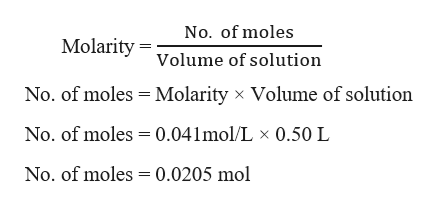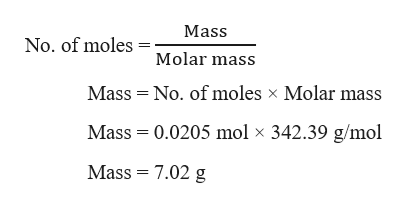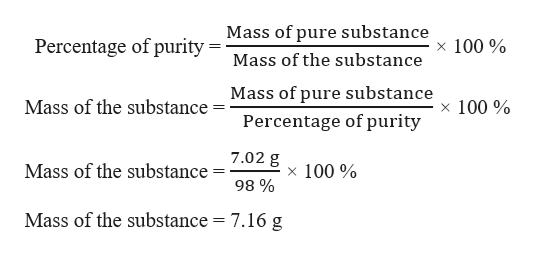# A tetraphenyl phosphonium chloride (TPPCl) powder (FW=342.39) is 98.0 percent pure. How many grams are needed to prepare 0.50 L of a 41.0 mM solution?

Question
93 views

A tetraphenyl phosphonium chloride (TPPCl) powder (FW=342.39) is 98.0 percent pure. How many grams are needed to prepare 0.50 L of a 41.0 mM solution?

check_circle

Step 1

Given,

Molar mass of tetraphenyl phosphonium chloride = 342.39 g/mol

Percentage of purity of tetraphenyl phosphonium chloride = 98.0 %

Molarity = 41.0 mM = 0.041 M

Volume = 0.50 L

Number of moles of tetraphenyl phosphonium chloride needed to prepare 0.50 L of a 0.041 M(mol/L) solution, can be calculated as:help_outlineImage TranscriptioncloseNo. of moles Molarity olume of solution No. of moles Molarity x Volume of solution No. of moles 0.041mol/L x 0.50 L No. of moles 0.0205 mol fullscreen
Step 2

Grams of tetraphenyl phosphonium chloride can be calculated as:help_outlineImage TranscriptioncloseMass No. of moles Molar mass Mass No. of moles x Molar mass Mass 0.0205 mol x 342.39 g/mol Mass 7.02 g fullscreen
Step 3

The calculated mass (7.02 g) is the pure mass of tetraphenyl phosphonium chloride required. But, it is given that purity of tetrapheny...help_outlineImage TranscriptioncloseMass of pure substance Percentage of purity =Mass of the substance x 100% Mass of pure substance Mass of the substance x 100 % Percentage of purity 7.02 g Mass of the substance x 100% 98 % Mass of the substance 7.16 g fullscreen

### Want to see the full answer?

See Solution

#### Want to see this answer and more?

Solutions are written by subject experts who are available 24/7. Questions are typically answered within 1 hour.*

See Solution
*Response times may vary by subject and question.
Tagged in

### General Chemistry